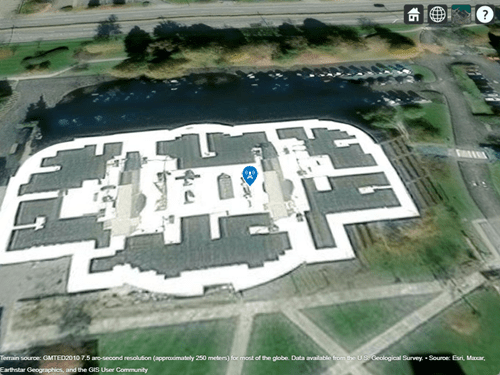# rxsite

## Description

Use the `rxsite` object to create a radio frequency receiver site.

## Creation

### Syntax

``rx = rxsite``
``rx = rxsite(Name,Value)``

### Description

example

````rx = rxsite` creates a radio frequency receiver site.```

example

````rx = rxsite(Name,Value)` sets properties using one or more name-value pairs. For example, ```rx = rxsite('Name','RX Site')``` creates a receiver site with name ```RX Site```. Enclose each property name in quotes.```

## Properties

expand all

Site name, specified as a character vector or as a row or column vector or as a string.

Example: `'Name','Site 3'`

Example: `RX.Name = 'Site 3'`

Example: If you want to assign multiple values then - ```names = ["Fenway Park","Faneuil Hall","Bunker Hill Monument"];`````` RX = rxsite('Name',names)```

Data Types: `char` | `string`

Site latitude coordinates, specified as a numeric scalar or a row or column vector in the range of range `-90` to `90`. Coordinates are defined using Earth ellipsoid model WGS-84. Latitude is the north/south angle.

Example: `'Latitude'`,`45.098`

Example: `RX = 45.098`

Example: If you want to assign multiple values then - ```latitude = [42.3467,42.3598,42.3763];`````` RX = rxsite('Latitude',latitude)```

Site longitude coordinates, specified as a numeric scalar or a row or column vector. Coordinates are defined using Earth ellipsoid model WGS-84. Longitude is the east/west angle.

Example: `'Longitude'`,`-68.890`

Example: `RX.Longitude = -68.890`

Example: If you want to assign multiple values then - ```longitude = [-71.0972,-71.0545,-71.0611];`````` RX = rxsite('Longitude',longitude)```

Antenna element or array specified as an object or `'isotropic'`. By default, the antenna is `'isotropic'`, which defines an antenna that radiates uniformly in all directions.

Example: `'Antenna'`,`monopole`

Example: ```TX.Antenna = monopole```

Antenna x-axis angle, specified as a numeric scalar, a 2-by-1 vector, or a 2-by-N matrix in degrees.

The numeric scalar is the azimuth angle measured counterclockwise from the east to the antenna x-axis.

In the 2-by-1 vector, the first element is the azimuth angle and the second element elevation angle. The elevation angle measures from the horizontal plane to antenna x-axis from `-90` to `90` degrees.

Example: `'AntennaAngle'`,`25`

Example: `RX.AntennaAngle = [25,-80]`

Data Types: `double`

Antenna height from the ground or building surface, specified as a non-negative numeric scalar in meters. Maximum value for this property is 6,371,000 m.

If the site coincides with the building, the height is measured from the top of the building to the center of the antenna. Otherwise,the height is measured from ground elevation to the center of the antenna.

Example: `'AntennaHeight'`,`25`

Example: `RX.AntennaHeight = 15`

Data Types:

System loss, specified as a non-negative numeric scalar or a row vector in dB.

System loss includes transmission line loss and any other miscellaneous system losses.

Example: `'SystemLoss'`,`10`

Example: `RX.SystemLoss = 10`

Data Types:

Minimum received power to detect the signal, specified as a numeric scalar or a row vector in dBm.

Example: `'ReceiverSensitivity'`,`-80`

Example: `RX.ReceiverSensitivity = -80`

Data Types: `double`

## Object Functions

 `show` Show site location on map `hide` Hide site location on map `distance` Distance between sites `angle` Angle between sites `elevation` Elevation of site `location` Location coordinates at a given distance and angle from site `sigstrength` Signal strength due to transmitter `los` Plot or compute the line-of-sight (LOS) visibility between sites on a map `link` Display communication link on map `pattern` Plot antenna radiation pattern on map

## Examples

collapse all

Create and show the default receiver site.

`rx = rxsite`
```rx = rxsite with properties: Name: 'Site 2' Latitude: 42.3021 Longitude: -71.3764 Antenna: 'isotropic' AntennaAngle: 0 AntennaHeight: 1 SystemLoss: 0 ReceiverSensitivity: -100 ```
`show(rx)`Create and show a 1-by-3 receiver site array using dipole antenna.

Define names and locations of the sites around Boston.

```names = ["Fenway Park","Faneuil Hall","Bunker Hill Monument"]; lats = [42.3467,42.3598,42.3763]; lons = [-71.0972,-71.0545,-71.0611];```

Define the sensitivity of the receivers.

` sens = -90;`

Create and show receiver site array.

```rxs = rxsite('Name', names,... 'Antenna',dipole, 'Latitude',lats,... 'Longitude',lons, ... 'ReceiverSensitivity',sens); show(rxs)```